# at

Execute chart at event broadcast or specified time

## Syntax

``at(n,E)``
``at(n,tick)``
``at(n,sec)``

## Description

example

````at(n,E)` returns `true` if the event `E` has occurred exactly `n` times since the associated state became active. Otherwise, the operator returns `false`.```

example

````at(n,tick)` returns `true` if the chart has woken up exactly `n` times since the associated state became active. Otherwise, the operator returns `false`.The implicit event `tick` is not supported when a Stateflow® chart in a Simulink® model has input events.```

example

````at(n,sec)` returns `true` if exactly `n` seconds have elapsed since the associated state became active. Otherwise, the operator returns `false`.In standalone charts in MATLAB®, specify `n` with a value greater than or equal to `0.001`. The operator creates a MATLAB `timer` object that generates an implicit event to wake up the chart. MATLAB `timer` objects are limited to 1 millisecond precision. For more information, see Events in Standalone Charts. The `timer` object is created when the chart finishes executing the `entry` actions of the associated state and its substates. If you specify `n` as an expression whose value changes during chart execution, the chart does not adjust the temporal delay of the `timer` object.The `timer` object starts running at the end of the chart step when the associated state becomes active. This step can include the execution of other parallel states in the chart.If the chart is processing another operation when it receives the implicit event from the `timer` object, the chart queues the event. When the current step is completed, the chart processes the event.If the state associated with the temporal logic operator becomes inactive before the chart processes the implicit event, the event does not wake up the chart. ```

Note

Using `at` as an absolute-time temporal logic operator is supported only in standalone charts in MATLAB. For charts in Simulink models, use the `after` operator instead. For more information, see Do Not Use at for Absolute-Time Temporal Logic in Charts in Simulink Models.

## Examples

expand all

Display a status message when the chart processes the third broadcast of the event `E` after the state became active.

``````on at(3,E): disp('ON');``````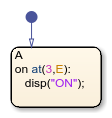Transition out of the associated state when the chart processes the fifth broadcast of the event `E` after the state became active.

`at(5,E)`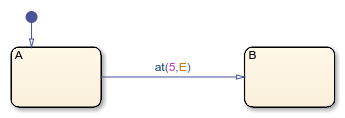Transition out of the associated state if the state has been active for exactly five broadcasts of the event `E`.

In charts in a Simulink model, enter:

`[at(5,E)]`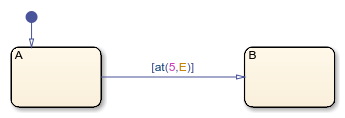Conditional notation for temporal logic operators is not supported in standalone charts in MATLAB.

Transition out of the associated state when the chart wakes up for the seventh time since the state became active, but only if the variable `temp` is greater than 98.6.

`at(7,tick)[temp > 98.6]`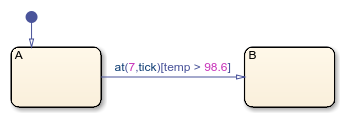Set the `temp` variable to `HIGH` if the state has been active for exactly 12.3 seconds.

In standalone charts in MATLAB, enter:

``````on at(12.3,sec): temp = HIGH;``````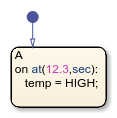Using `every` as an absolute-time temporal logic operator is not supported in charts in Simulink models.

## Tips

• You can use quotation marks to enclose the keywords `'tick'` and `'sec'`. For example, `at(5,'tick')` is equivalent to `at(5,tick)`.

• The Stateflow chart resets the counter used by the `at` operator each time the associated state reactivates.

• Standalone charts in MATLAB define absolute-time temporal logic in terms of wall-clock time, which is limited to 1 millisecond precision.